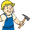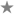Documentation de modèle (Pour le modèle ci-dessus, parfois caché ou invisible)

## Sommaire

Modèle auxiliaure à utiliser dans la section statistiques dérivées du modèle `{{Infobox personnage}}`.

## Utilisation

Placez les lignes suivantes dans le paramètre `|derivées =` des infobox personnage :

```{{Stats dérivées
<!-- insérez les paramètres -->
}}
```

### ParamètresTraduction en cours Cet article ou cette section a besoin d'être traduit(e). Merci d'aider l'Abri en améliorant cet article.

#### Paramètres stats dérivéesap
Base level Action Points.ap max
Action Points at highest level.ac
Base level Armor Class.ac max
Armor Class at highest level.carry weight
Base level Carry Weight.carry weight max
Carry Weight at highest level.crit chance
Base level Critical Chance.crit chance max
Critical Chance at highest level.dt
Base level Damage Threshold.dt max
Damage Threshold at highest level.dr
Base level Damage Resistance.dr max
Damage Resistance at highest level.er
Base level Energy Resistance.er max
Energy Resistance at highest level.fatigue
Base level Fatigue.fatigue max
Fatigue at highest level.fire resist
Base level Fire Resistance.laser resist
Base level Laser Resistance.plasma resist
Base level Plasma Resistance.explosion resist
Base level Explosion Resistance.electricity resist
Base level Electricity Resistance.fire resist max
Fire Resistance at highest level.hp
Base level Hit Points.hp max
Hit Points at highest level.melee damage
Base level Melee Damage.melee damage max
Melee Damage at highest level.poison resist
Base level Poison Resistance.poison resist max
Poison Resistance at highest level.unarmed damage
Base level Unarmed Damage.unarmed damage max
Unarmed Damage at highest level.sequence
Base level Sequence.sequence max
Sequence at highest level.healing rate
Base level Healing Rate.healing rate max
Healing Rate at highest level.perk rate
Base level Perk Rate.perk rate max
Perk Rate at highest level.skill rate
Base level Skill Rate.skill rate max
Skill Rate at highest level.experience points
Experience Points.

#### Derived stats modifier parametersacmod
Armor Class modifier applied to derived stats. Can be a negative number.agmod
Agility modifier applied to derived stats. Can be a negative number.ccmod
Critical % Multiplier modifier applied to derived stats. Can be a negative number.cwmod
Carry Weight modifier applied to derived stats. Can be a negative number.dtmod
Damage Threshold modifier applied to derived stats. Can be a negative number.drmod
Damage Resistance modifier applied to derived stats. Can be a negative number.enmod
Endurance modifier applied to derived stats. Can be a negative number.frmod
Fire Resistance modifier applied to derived stats. Can be a negative number.lrmod
Laser Resistance modifier applied to derived stats. Can be a negative number.prmod
Plasma Resistance modifier applied to derived stats. Can be a negative number.exrmod
Explosion Resistance modifier applied to derived stats. Can be a negative number.elrmod
Electricity Resistance modifier applied to derived stats. Can be a negative number.lkmod
Luck modifier applied to derived stats. Can be a negative number.stmod
Strength modifier applied to derived stats. Can be a negative number.udmod
Unarmed Damage modifier applied to derived stats. Can be a negative number.

## Exemple

```{{Stats dérivées
|ap                 =90
|ap max             =120
|agmod              =1
|carry weight       =150
|carry weight max   =200
|hp                 =90
|enmod              =1
|melee damage       =15
}}
```
Renders
 Points de vie : 90  (95) Points d'action : 90→120 (93→123)

### Copy/paste

```{{Derived stats
|ac                 =
|ac max             =
|ap                 =
|ap max             =
|carry weight       =
|carry weight max   =
|crit chance        =
|crit chance max    =
|dr                 =
|dr max             =
|dt                 =
|dt max             =
|fatigue            =
|fatigue max        =
|fire resist        =
|fire resist max    =
|hp                 =
|hp max             =
|melee damage       =
|melee damage max   =
|poison resist      =
|poison resist max  =
|unarmed damage     =
|unarmed damage max =
|sequence           =
|healing rate       =
|skill rate         =
|perk rate          =
|experience points  =
|acmod              =
|agmod              =
|ccmod              =
|cwmod              =
|drmod              =
|dtmod              =
|enmod              =
|frmod              =
|lkmod              =
|prmod              =
|exrmod             =
|elrmod             =
|stmod              =
|udmod              =
}}
```
Documentation extraite depuis Modèle:Stats dérivées/doc.